Home | | Engineering Physics II | Conducting Materials

# Conducting Materials

1 Introduction 1.1.Basic Terminologies 2 Conducting Materials 3 Electron Theory of solids 4 Classical Free Electron Theory 4.1. Drude – Lorentz Theory 4.2. Postulates of Classical Free Electron Theory 4.3. Derivation of Electrical conductivity 4.4. Thermal Conductivity 5 Wiedemann - Franz Law 6 Lorentz Number 7 Merits of classical free electron theory 8 Drawbacks of classical free electron theory 9 Quantum Free Electron Theory 10 Fermi Dirac Distribution Function 10.1. Effect of Temperature 11 Density of Energy States 11.1. Definition 11.2. Derivation 11.3. Calculation of Carrier Concentration at 0 K 11.4. Calculation of Fermi Energy 11.5. Average Energy of an Electron at 0 K

CONDUCTING MATERIALS

1 Introduction

1.1.Basic Terminologies

2 Conducting Materials

3 Electron Theory of solids

4 Classical Free Electron Theory

4.1. Drude – Lorentz Theory

4.2. Postulates of Classical Free Electron Theory

4.3. Derivation of Electrical conductivity

4.4. Thermal Conductivity

5 Wiedemann - Franz Law

6 Lorentz Number

7 Merits of classical free electron theory

8 Drawbacks of classical free electron theory

9 Quantum Free Electron Theory

10 Fermi Dirac Distribution Function

10.1. Effect of Temperature

11 Density of Energy States

11.1. Definition

11.2. Derivation

11.3. Calculation of Carrier Concentration at 0 K

11.4. Calculation of Fermi Energy

11.5. Average Energy of an Electron at 0 K

1 INTRODUCTION

Conducting materials are low resistivity materials, which conduct heat as well as electricity. Electrical conduction is due to free electrons, whereas normal conduction is due to free electrons as well as phonons.

1.1. BASIC TERMINOLOGIES

Conductors

Experimental measurements showed that the metals and their alloys exhibit large electrical conductivity in the order of 10 Ω m . Hence they are known as conductors Conducting materials are the materials having high electrical and thermal conductivities. Low resistive materials are also generally known as conducting materials.

Bound Electrons

All the valence electrons in an isolated atom are bound to their parent nuclei which are called as ‘ bound electrons’

Free Electrons

In a solid, due to the boundaries of neighbouring atoms overlap each other, the valence electrons find continuity from atom to atom. Therefore, they can move easily throughout the solid. All such valence electrons of its constituent atoms in a solid are called free electrons.

Difference Between Ordinary Gas and Free Electron Gas

The molecules of ordinary gas are neutral. But, the free electron gas is charged. The density of molecules is smaller than the density of free electrons.

Electric Field (E)

The electric field (E) of a conductor having uniform cross section is defined  as the potential

drop (V) per unit length (l).

E = V/ l

Unit –Vm-1

Current Density (J)

Current density (J) is defined as the current per unit area of cross section of an imaginary plane hold normal to the direction of flow of current in a current carrying conductor.

If       ‘I’   is   the   current,   and   ‘A’   is   the   area   of

J = I / A      Unit –A m-2

2 CONDUCTING MATERIALS

Conducting materials are classified in to three major categories based on the conductivity.

(i). Zero resistive materials (ii). Low resistive materials (iii). High resistive materials

(i)Zero resistive materials

The super conductors like alloys of aluminium, zinc, gallium, niobium, etc., are a special class

of materials. These materials conduct electricity almost with zero resistance blow transition temperature. Thus, they are called zero resistive materials.

These materials are used for saving energy in the power systems, super conducting magnets, memory storage elements etc.,

(ii)Low resistive materials

The metals like silver, aluminium and alloys have high electrical conductivity. These materials are called low resistive materials.

They are used as conductors, electrical co

nduct etc., in electrical devices and electrical power transmission and distribution, winding wires in motors and transformers.

(iii)High resistive materials

The materials like tungsten, platinum, nichrome etc., have high resistive and low temperature co-efficient of resistance. These materials are called high resistive materials.

Such a metals and alloys are used in the manufacturing of resistors, heating elements, resistance thermometers.

The conducting properties of solid do not depend on the total number of the electrons available because only the valance electrons of the atoms take part in the conduction. When these valance electrons detached from the orbit they are called free electrons or conduction electrons.

In a metal, the number of free electrons available is proportional to its electrical conductivity. Hence, electronic structure of a metal determines its electrical conductivity.

3 ELECTRON THEORY OF SOLIDS

We know that the electrons in the outermost orbit of the atom determine the electrical properties in the solid. The free electron theory of solids explains the structure and properties of solids through their electronic structure.

This theory is applicable to all solids, both metals and non metals. It explains

(i). The behavior of conductors, semiconductors, and insulators. (ii). The electrical, thermal and magnetic properties of solids.

So far three electron theories have been proposed.

(i). Classical free electron theory

It is a macroscopic theory, proposed by Drude and Lorentz in1900. According to this theory, the free electrons are mainly responsible for electrical conduction in metals. This theory obeys laws of classical mechanics.

(ii). Quantum free electron theory

It is a microscopic theory, proposed by sommerfeld in 1928. According to this theory, the electrons in a metals move in a constant potential. This theory obeys laws of quantum mechanics.

(iii). Zone theory or band theory of solids

Bloch proposed this theory in the year 1928. According to this theory, the free electrons move in a periodic potential. This theory explains electrical conductivity based on the energy bands.

4 CLASSICAL FREE ELECTRON MODEL

Free electron theory of metals was proposed by P. Drude in the year 1900 to explain electrical conduction in metal. This theory was further extended by H.A. Lorentz in the year 1909.

4.1. DRUDE LORENTZ THEORY

Principle

According to this theory, a metal consists of a very large number of free electrons. These free electrons can move freely throughout the volume of the metal. They are fully responsible for the electrical conduction in the metal.

Explanation

We know that an atom consists of a central nucleus with positive charge surrounded by the electrons of negative charge. The electros in the inner shells are called core electros and those in the outermost shell are called valence electrons.4.2.POSTULATES OF FREE ELECTRON THEORY

Drude assumed that the free electrons in a metal form an electron gas. These free electrons move randomly in all possible directions just like the gas molecules in a container.

In the absence of electrical field

When an electrical field is not applied, the free electrons move everywhere in a random manner. They collide with other free electrons and positive ion core. This collision is known as elastic collision.In the presence of electric field

When the electrical field is applied, the electrons get some amount of energy from the applied electric field and they begin to move towards the positive potential. (In the opposite direction to the applied electric field).Since electrons are assumed to be a perfect gas, they obey the laws of kinetic theory of gases.

Drift velocity (v d)

It is defined as the average velocity acquired by the free electrons in a metal in a particular direction by the application of an electrical field.

Mean free   path   (λ)

The average distance travelled by a free electron between any two successive collisions in the presence of an applied electric field is known as mean free path. It is the product of drift velocity of free electron and collision time ( ι c).Collision time ( ι c)

The average time taken by a free electron between any two successive collisions is known as collision time. It is expressed mathematically asRelaxation time   (ι)

The average time taken by a free electron to reach its equilibrium position from its disturbed position due to the application of an external electrical field is called relaxation time. It is approximately equal to 10-14 second.

4.3 DERIVATION OF ELECTRICAL CONDUCTIVITY

Definition

The amount of electrical charge conducted (Q) per unit time across unit area (A) of a solid given by

σ        =   Q   /   tAE

If  t = 1second E = 1 volt, A = 1 metre 2

σ        =   Q

σ = Q / tAE = J / E

Where J is the current density and it is given by Q / tA. Also J = σ E ( according to ohm’s law )

Expression for electrical conductivity

We know in the absence of external electric field, the motion of electrons in a metal moves randomly in all directions. When electric fi rod, the electron moves in opposite direction to the applied field with velocity v d. this velocity is known as drift velocity.Lorentz force acting on the electron F = eE   --- (1)

This force is known as driving of the electron. Due to this force, the electron gains acceleration ‘a’. From motion, the Newton’s force second law of

F= ma  --- (2)

From equations 1 & 2

ma = eE(or)

a = eE / m  -- (3)

The acceleration of electron is given by

Acceleration (a) = Drift velocity (v d) / Relaxation time   (τ)

a = v d / τ

v d =   a τ

Substituting equation (3) in( 4)

v d  =[   e   τ   /   m   ]   E   ---(5)

Where ‘σ’ is the electrical conductivity of velocity is given as

J = ne v d   --(6)

Substituting equation (5)in (7), we have

J = ne [e τ / m] E              ---(7)

J = [ne2 τ / m] E , in terms of effective mass m* of an electron,

J = [ne2 τ / m* ] E      ---(8)

From microscopic form of Ohm’s law, the current density ‘J’ is expressed as,

J = σ E            ---(9)

On comparing equations (8) & (9) , we have

Electrical conductivity σ = ne2 τ / m*

From equation (10), we know that with increase of electron concentration ‘n’ , the conductivity ‘σ’ increases. As m* increases, the motion of electron becomes slow and the electrical conductivity.

4.4.THERMAL CONDUCTIVITY

Definition

It is defined as the amount of heat flowing per unit time through the material having unit area of cross section per unit temperature gradient.

ie)., Q = K dT/ dx

Thermal conductivity of the material

K = Q / dT/dx

Q –Amount of heat flowing per unit time through unit cross sectional area

Expression for thermal conductivity

Let us consider a uniform rod AB with temperatures T1 (Hot) at end A and T2 (cold) at end B. Heat flows from hot end A to the hold end B. Let us consider cross sectional area C which is at a distance equal to the mean free path (λ) of t in fig.The conduction electron per unit value is n and average velocity of these electrons is v.

During the movement of electrons in the rod, the collision takes place. Hence, the electrons near A lose their kinetic energy while electrons near B gain kinetic energy.

At A, average kinetic energy of en electron = 3/2 kT            ------(1)

Where k- Boltzmann’s   constant   and   T   is   temperature

At B average kinetic energy of the electron = 3/2 k (T –dT)           ------(2)

The excess of kinetic energy carried by the electron from A to B

= 3/2 kT - 3/2 k (T –dT)

= 3/2 kT - 3/2 kT + 3/2 k dT          ------(3)

Number of electrons crossing per unit area per unit time from A to B

= 1/6 nv.

The excess of energy carried from A to B per unit area in unit time

= 1/6 nv X 3/2 kdT

= 1/4 n v k dT             ------(4)

Similarly, the deficient of energy carried from B to A per unit area per unit time

= -1/4 n v kdT             ------(5)

Hence, the net amount of energy transferred from A to B per unit area per unit time

Q =1/4 nvkdT –(-1/4 nvkdT )

Q = 1/4 nvkdT + 1/4 nvkdT

Q = 1/2 nvkdT            ------(6)

But from the basic definition of thermal conductivity, the amount of heat conducted per unit area per unit time

Q      =   K   dT/   λ

[        Q   =   K   dT/   dx   ;     λ   =   dx]

½ n   u   k   dT     = K   dT/   λ

K = ½ nv k λ (7)

We know that for the metals

Relaxation time        =      collision time

τ = τ c = λ / v

τ v = λ     ---(8)

Substituting equation 8 in the equation 7, we have

K = ½ n v k τ v

K = ½ n v2 k τ    ---(9)

5 WIDEMANN –FRANZ LAW

Statement

It states that for the metals, the ratio of thermal conductivity to electrical conductivity is directly proportional to the absolute temperature. This ratio is a constant for all metals at given temperature.

K / σ   ∞   T

Or

Where L is a proportionality constant. It is known as Lorentz number. Its value is 2.44 X 10-8

-2

WΩK at T = 293 K.

Derivation

Widemann –Frantz law is derived from the expressions of thermal conductivity and electrical conductivity of metal.

We know that,

Electrical conductivity of a metal σ = ne2τ / m    ----(1)

Thermal conductivity of a metal K = ½ n v2k τ    ----(2)

Thermal conductivity   =  ½ n v2 k τ

Electrical conductivity  = ne2 τ   /   m

K / σ  = ½  mv2 k / e2     ---(3)

We know that the kinetic energy of an electron

Or       ½  mv2 = 3/2 kT         ---(4)

Substituting equation 4 in equation 3, we have

K / σ = 3/2 kT  X   k / e2

= 3/2 [k/e] 2 T

K / σ = LT             ---(5)

where L = 3/2 [k/e] 2 is a constant and it is known as Lorentz number

K / σ = αT    ----(6)

Thus it is proved that, the ratio of thermal conductivity to electrical conductivity of a metal is directly proportional to the absolute temperature of the metal.

Conclusion

Wiedamann –Franz law clearly shows that if a metal has high thermal conductivity, it will also have high electrical conductivity.

6 LORENTZ NUMBER

The ratio of thermal conductivity (K) of of the metal and absolute temperature (T) of the metal is a constant. It is known as Lorentz number

and it is given by

L    =   K   /   σ

7MERITS OF CLASSICAL FREE ELECTRTON THEORY

1.     It   is   used   to   verify   Ohm’s   Law.

2.     The electrical and Thermal conductivities of metals can be explained by this theory.

3.     It is used to derive Wiedemann- Franz law.

4.     It is used to explain the optical properties of metals.

8 DRAWBACKS OF CLASSICAL FREE ELECTRON THEORY

It is a macroscopic theory

Classical theory states that all free electrons will absorb energy, but quantum theory states that only few electrons will absorb energy.

This theory cannot explain the Compton effect, photoelectric effect, paramagnetism, ferromagnetism etc.,

The theoretical and experimental values of specific heat and electronic specific heat are not matched.

By classical theory Kratures,/σT= butconstantbyquanfo constant for all temperatures.

The Lorentz number by classical theory does not have good agreement with experimental value and it is rectified by quantum theory.

9 QUANTUM THEORY

The drawbacks of classical theory can be rectified using quantum theory. In classical theory the properties of metals such as electrical and thermal conductivities are well explained on the assumption that the electrons in the metal freely moves like the particles of gas and hence called free electron gas.

According to classical theory, the particles of gas (electrons) at zero Kelvin will have zero kinetic energy, and hence all the particles are at rest. But according to quantum theory when all particles at rest, all of them should be filled only in the ground state energy level, which is impossible and is controversial to the pauli’s exclusion principle.

Thus in order to fill the electrons in a given energy level, we should know the following. Energy distribution of electrons

Number of available energy states Number of filled energy states

Probability of filling an electron in a given energy state, etc.,

10 FERMI DIRAC DISTRIBUTION FUNCTION

The classical and quantum free electron theories failed to explain many electrical and thermal properties of solids. However, these properties can be easily understood using Fermi –Dirac statistics.

Fermi –Dirac statistics deals with the particles having half integral spin. The particles like electrons are the examples of half integral spin and hence they are known as Fermi particles or Fermions.

Definition

The expression which gives the distributions of electrons among the various energy levels as a function of temperature is known as Fermi distribution function. It is the probability function F(E) of an electron occupying given energy level at absolute temperature. It is given by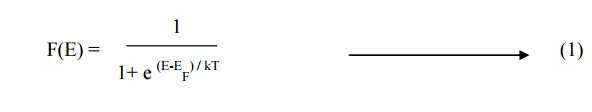Where

E –Energy of the level whose occupancy is being considered

EF –Energy of the Fermi level

K –Boltzmann’s   constant

T –Absolute temperature

The probability value of F(E) lies between 0 and 1. If F(E) = 1, the energy level is occupied by an electron. If F(E) = 0, the energy level is vacant. If F(E) = ½ or 0 .5 then there is a 50% chance for the electron occupying in that energy level.

Effect of temperature on Fermi function

The effect of temperature on Fermi function F(E) can be discussed with respect to equation 1.

1.  At 0 kelvin

At 0 kelvin, the electron can be filled only upto a maximum energy level called Fermi energy level (EF0), above EF0 all the energy levels will be empty. It can be proved from the following conditions.

When E<EF, equation 1 becomes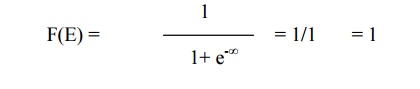(i.e 100% chance for the electron to be filled within the Fermi energy level)

When E>EF, equation 1 becomes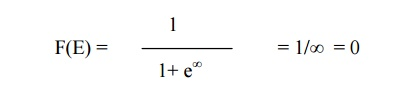(i.e zero% chance for the electron not to be filled within the Fermi energy level)

When E = EF, equation 1 becomes(i.e 50% chance for the electron to be filled within the Fermi energy level)

This clearly shows that at 0 kelvin all the energy states below EF0 are filled and all those above it are empty.

The Fermi function at 0 kelvin can also be represented graphically as shown in fig .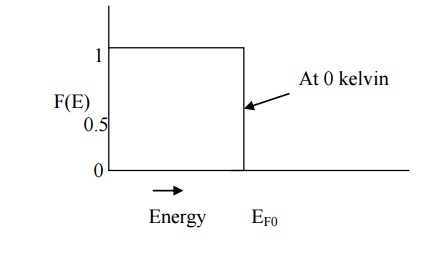Fermi energy and its importance Fermi energy level

Fermi energy level is the maximum energy level upto which the electron can be filled at ok. Importance:

Thus it act as a reference level which separates the vacant and filled energy states at 0k. It gives the information about the filled electron states and the empty states.

At 0k, blow Fermi energy level electrons are filled and above Fermi energy level it will be empty.

When the temperature is increased, few electrons gains thermal energy and it goes to higher energy levels.

Conclusions:

In the quantum free electron theory, though the energy levels are descrete, the spacing between consecutive energy level is very less and thus the distribution of energy levels seems to be continuous.

The number of energy levels N(E) that are filled with electrons per unit energy increases parabolic ally with increase of energy E as shown in fig.Each energy level can provide only two states, namely, one for spin up and other for spin down and hence only two electrons can be exclusion principle.

At T = 0, if there are N number of atoms, then we have N/2 number of filled energy levels and other higher energy levels will be completely empty.

This (N/2) th energy level is the highest filled energy level is known as Fermi energy level (EFO).

The electrons are filled in a given energ no two electrons can have the same set of four quantum numbers.

At room temperature, the electrons within the range of KBT below the Fermi energy level will absorb thermal energy = KBT and goes to higher energy states with energy EF0 + KBT.

11 DENSITY OF STATES

The Fermi function F(E) gives only the probability of filling up of electrons on a given energy state, it does not give the information about the number of electrons that can be filled in a given energy state. To know that we should know the number of available energy states so called density of states.

11.1.Definition

Density of states Z(E)dE is defined as the number of available electron states per unit volume in an energy interval (dE).

Explanation

In order to fill the electrons in an energy state we have to first find the number of available energy states within a given energy interval.

We know that a number of available energy levels can be obtained for various combinations of quantum numbers nx, ny, and nz . (i.e) n2 = nx2 + ny2 + nz2

Therefore, let us construct three dimensional space of points which represents the quantum numbers nx, ny, and nz as shown in fig. in this space each point represents an energy level.11.2.DERIVATION OF DENSITY OF ENERGY STATES

To find the number of energy levels in a cubical metal piece and to find number of electrons that can be filled in a given energy level, l

The sphere is further divided in to many shells and each of this shell represents a particular combination of quantum numbers (nx, n y, and nz) and therefore represents a particular energy value.

Let us consider two energy values E and E + dE. The number of energy states between E and E + dE can be found by finding the number of energy states between the shells of the radius n and n + Δn, from the origin.

The number of energy states within the sphere of radius  n = 4/3 πn3

Since nx, ny, and nz will have only positive values, we have to take only one octant of the sphere (i.e) 1/8 th of the sphere volume.

The number of available energy states within the sphere of radius n = 1/8 [4/3 π n3]

Similarly the number of available energy states within the sphere of radius n + dn

n + dn = 1/8 [4/3 π (n+dn)3 ]

The number of available energy states between the shells of radius n and n + dn (or) between the energy levels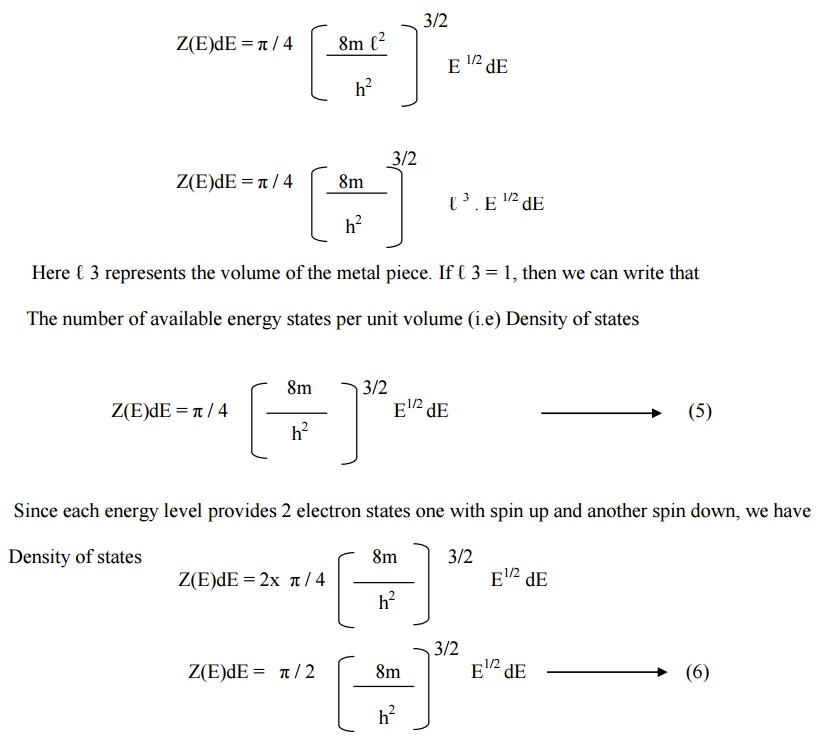11.3. CALCULATION OF CARRIER CONCENTRATION

Let N(E) dE represents the number of filled energy states between the interval of energy dE . Normally all the energy states will not be filled. The probability of filling of electrons in a given energy state is given by Fermi function F(E).

N(E) = Z(E)dE . F(E)    ----(7)

Substituting equation (6) in equation (7), we get

Number of filled energy states per unit volume

N(E) = π / 2 [8m / h2 ] E ½ dE. F(E)          ----(8)

N(E) is known as carrier distribution function (or) carrier concentration in metals.

11.4.CALCULATION OF FERMI ENERGY

Case (i) At T= 0KCase(ii) At T> 0K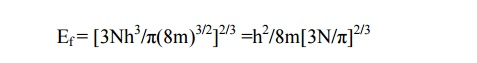11.5.AVERAGE ENERGY OF AN ELECTRON AT OK

Average energy =  Total energy / Carrier ConcentrationEavg= 3/5 Efo

Study Material, Lecturing Notes, Assignment, Reference, Wiki description explanation, brief detail
Physics : Conducting Materials : Conducting Materials |